DIY

# Electronic memory training device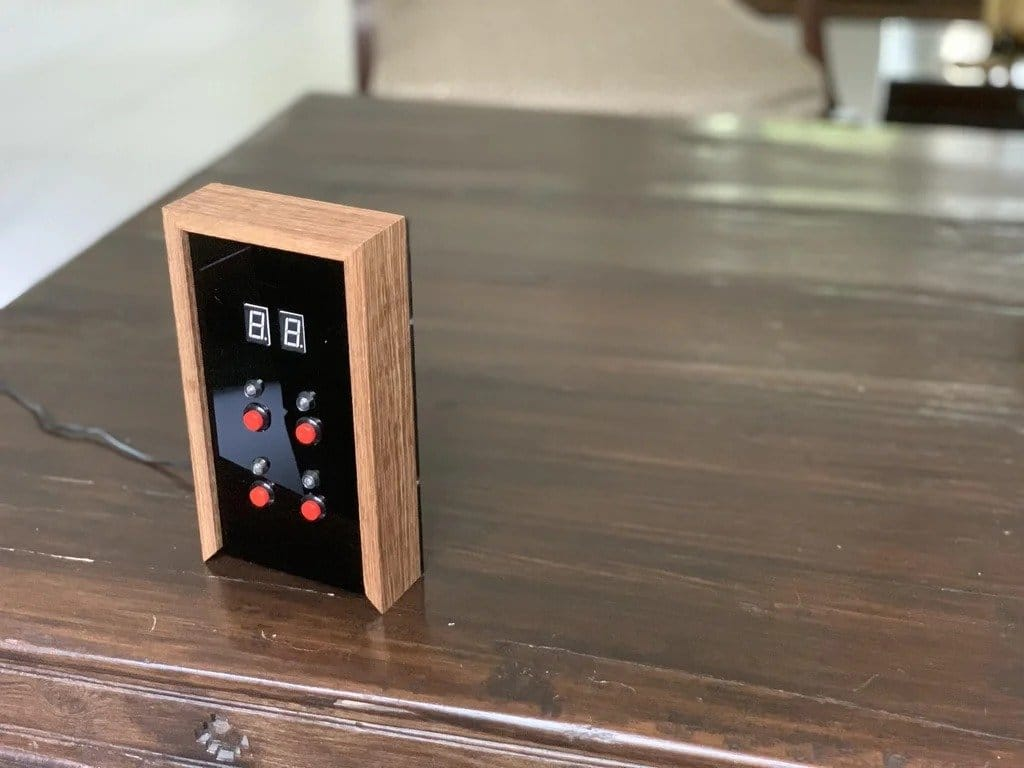This device is a panel with four buttons, four LEDs and a digital display.
The LEDs light up in a random order, and the user must press the buttons in the same order. Each time (level) the difficulty increases, and the level is displayed on the digital display.
How it works can be seen on the video.

Tools and materials: -4 buttons; -12 resistors 10 kOhm; -18 resistors of 470 Ohm;
-8 diodes;
-7-segment display -2 pcs; -Two integrated circuits 4511; -Mounting board; -Power adapter 5 V; -Arduino Nano;
-Extension board Arduino Nano;
-LEDs -4 pcs;
-Drilling machine;
-Miter saw; -Clips; -Laser cutter; -Soldering iron; -Stripper; – Computer; -PVA;
-Wood;
-Fasteners;
-Black acrylic;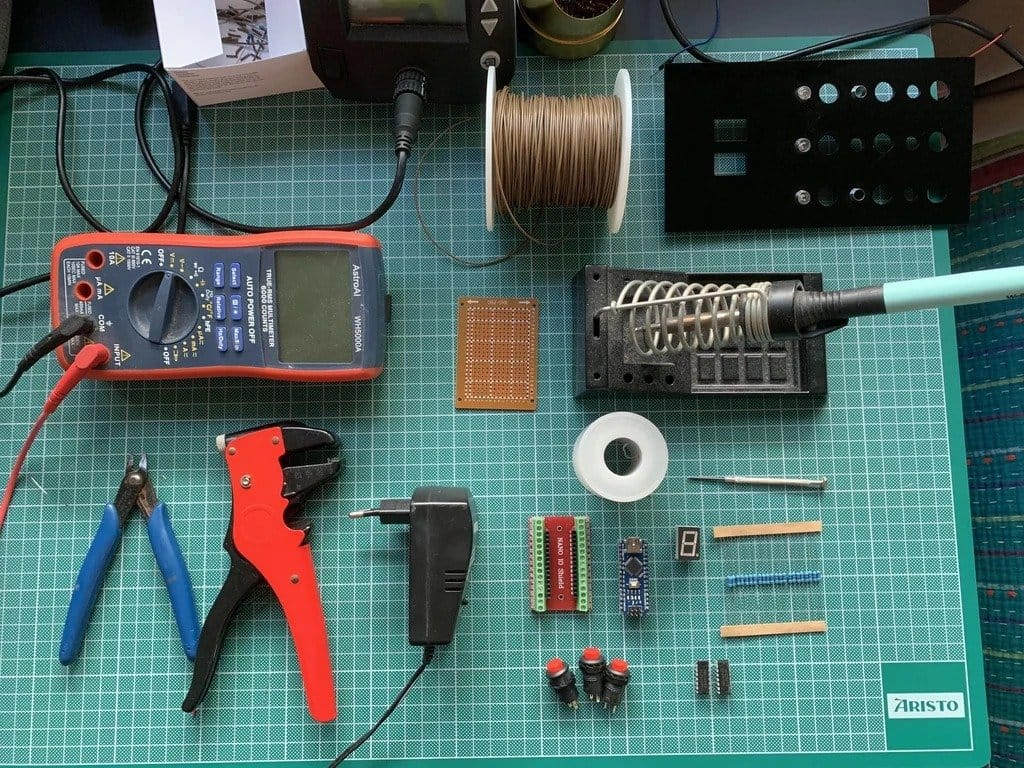Step one: model development
First, the wizard developed a device design in the Fusion 360 program. For the case the master chose teak wood, and for the front panel – black acrylic.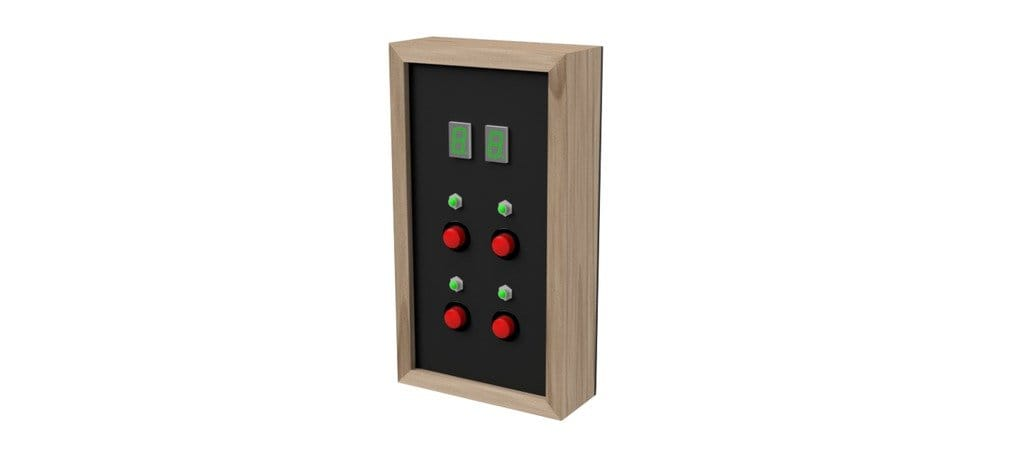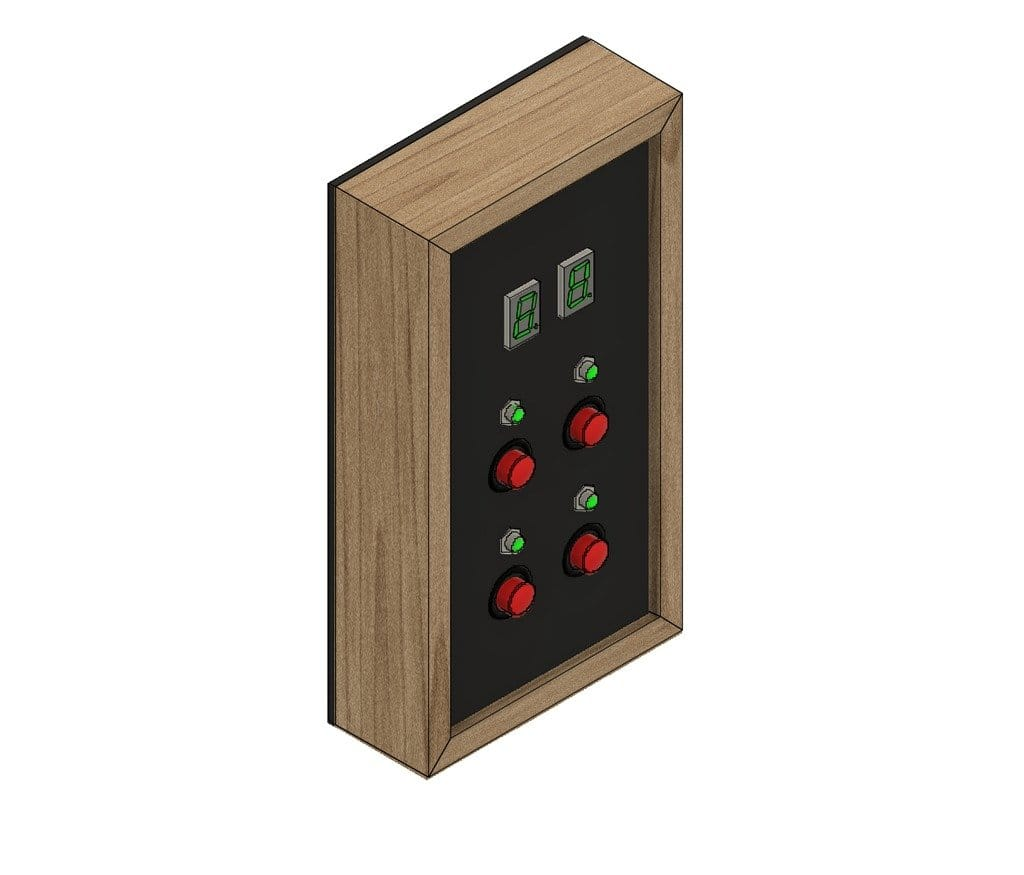Step two: corpus
Next, you need to make the body. As mentioned earlier, the body material is teak wood. The slats are 10 mm thick and 40 mm wide. Sawing parts according to the drawings.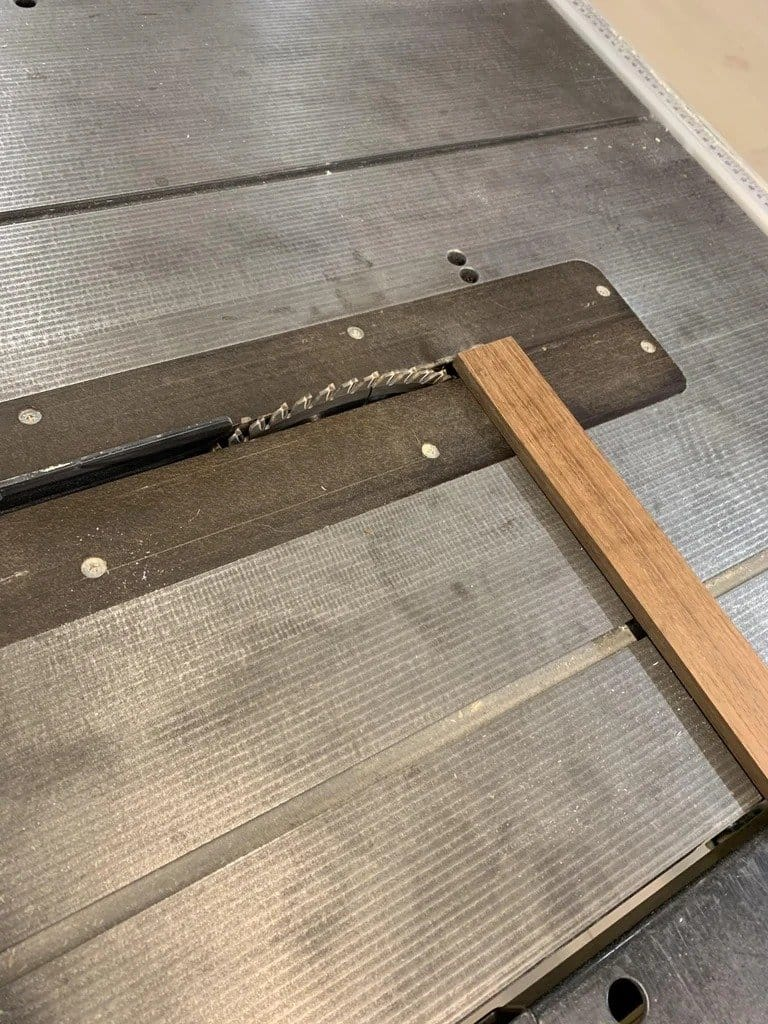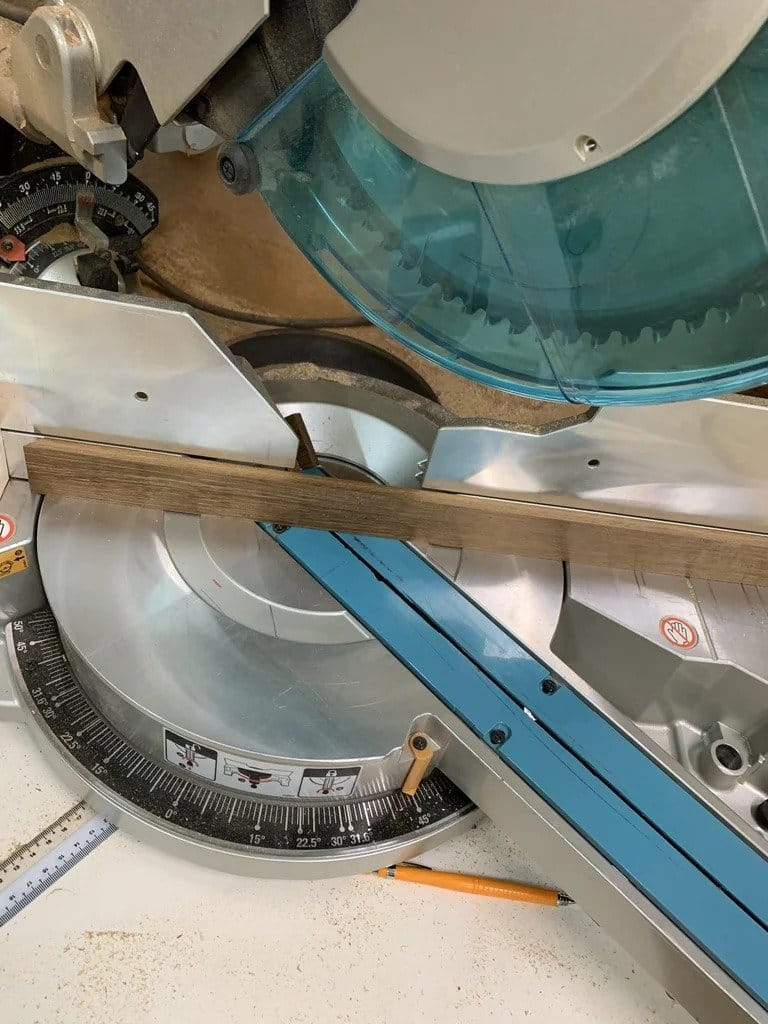Cut grooves for the acrylic plate in the strips.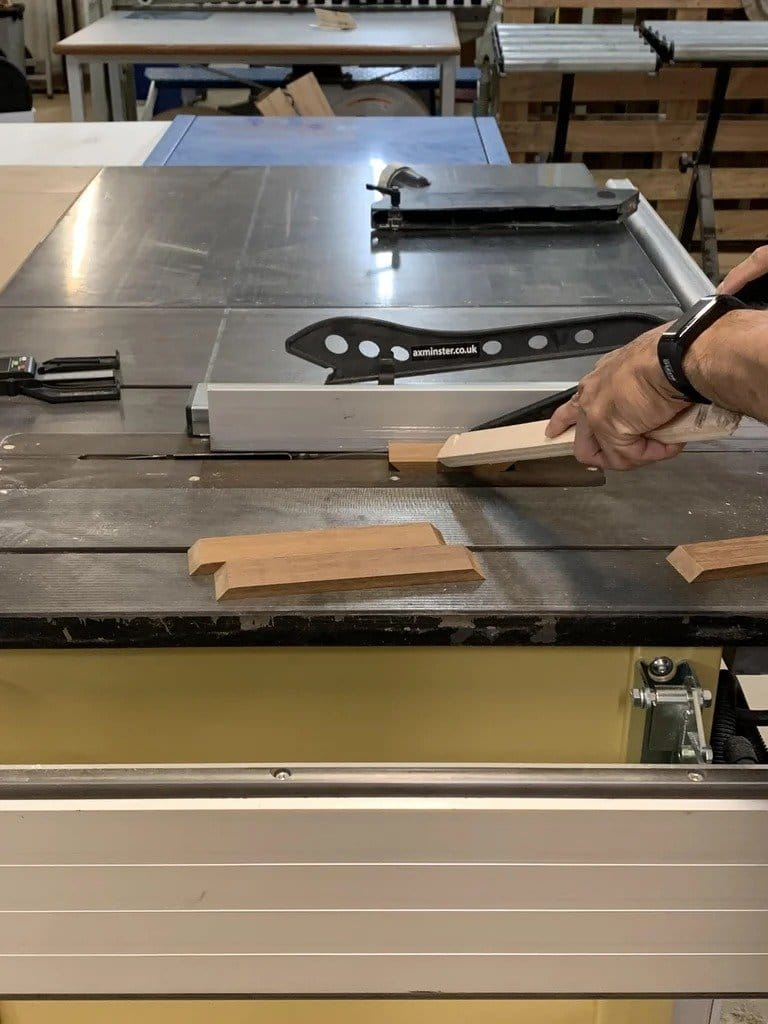Bonds body parts, except for one upper bar.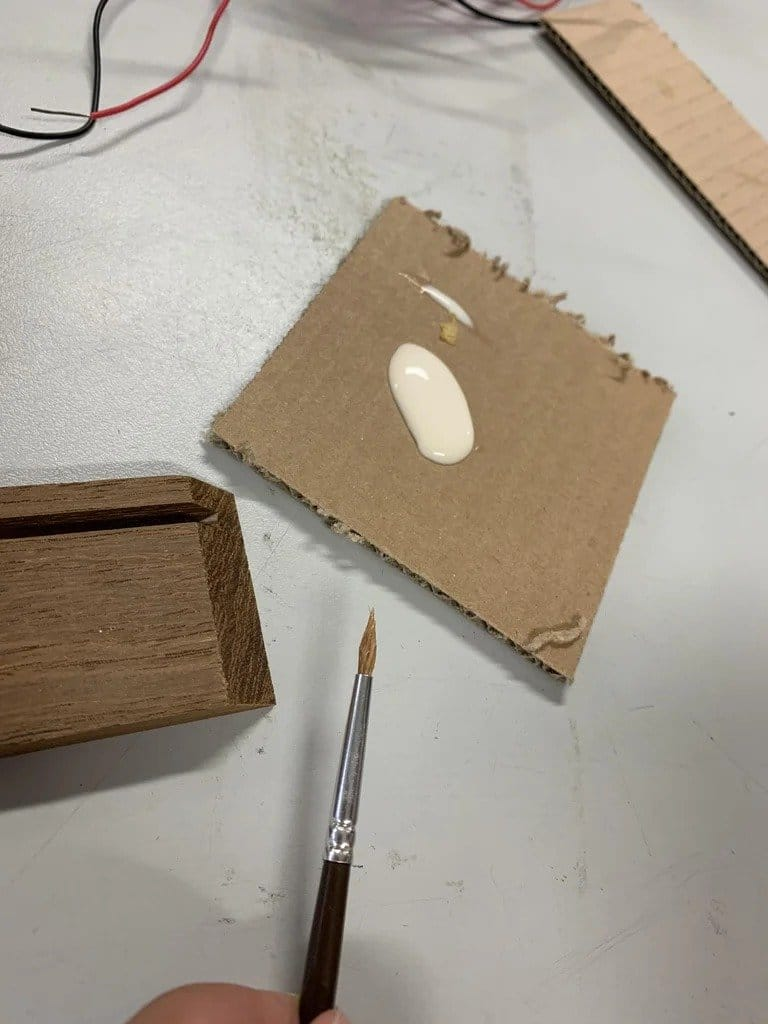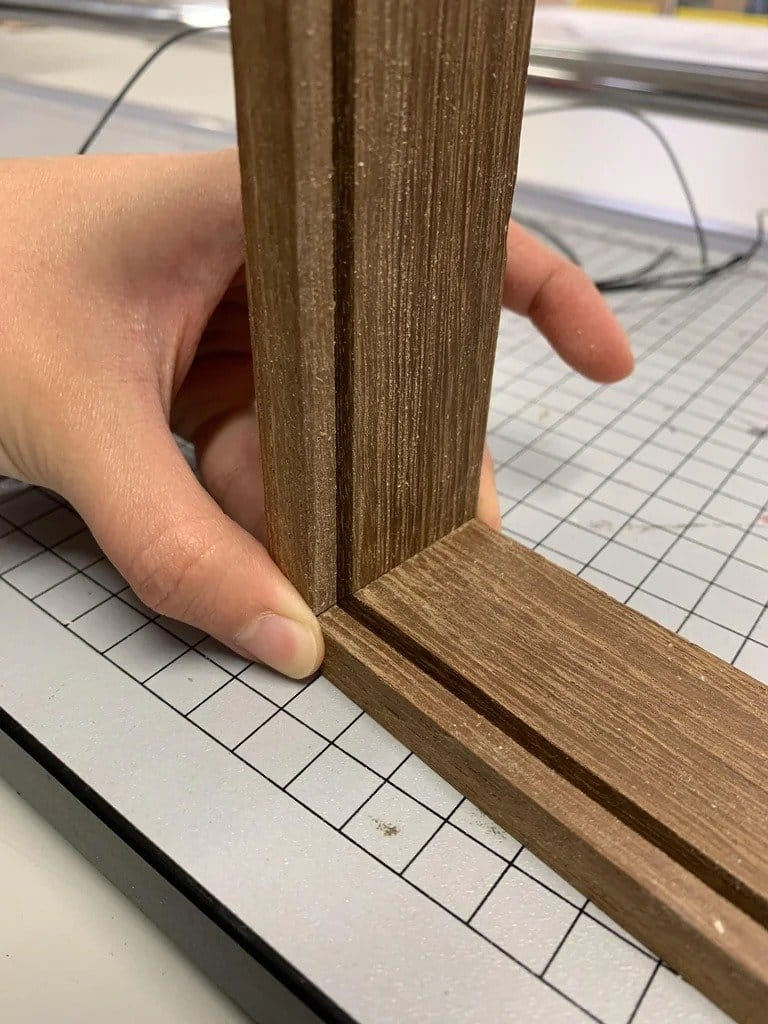Step three: laser cutting
Holes in the front panel (for buttons, LEDs and display) and holes in the top panel (for fasteners) are cut by the master on a laser cutter.
Cutting files included.
UI.svgTestCut.svg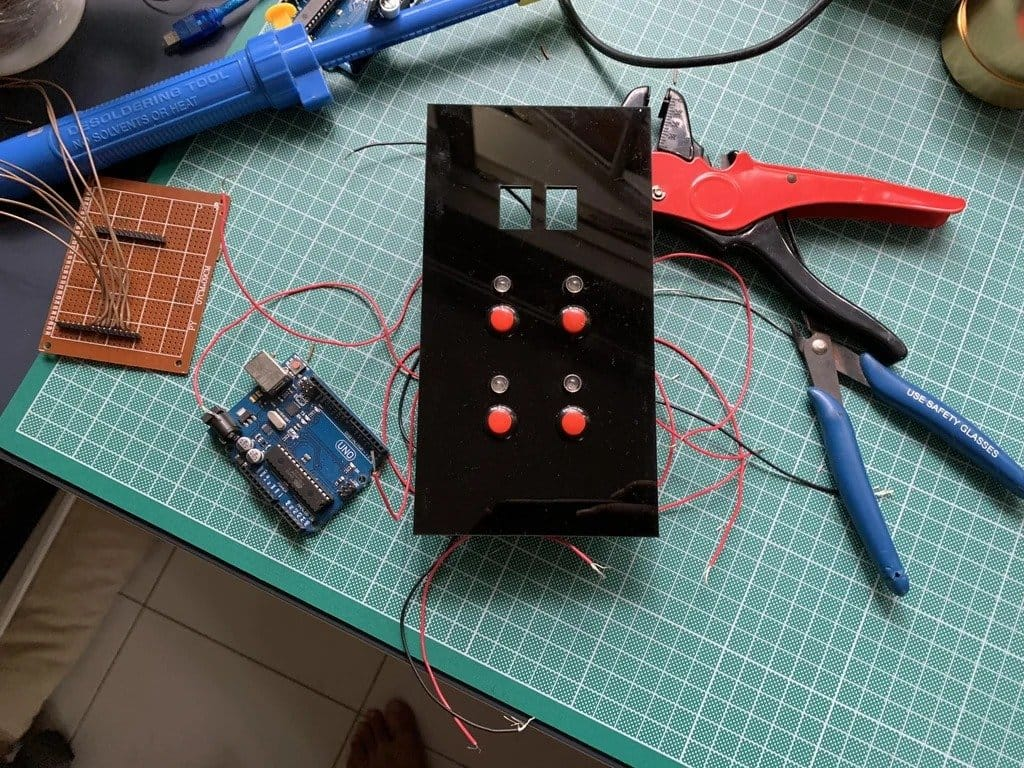Back acrylic plate simply screwed to the body with screws. To do this, you need to drill four holes in it.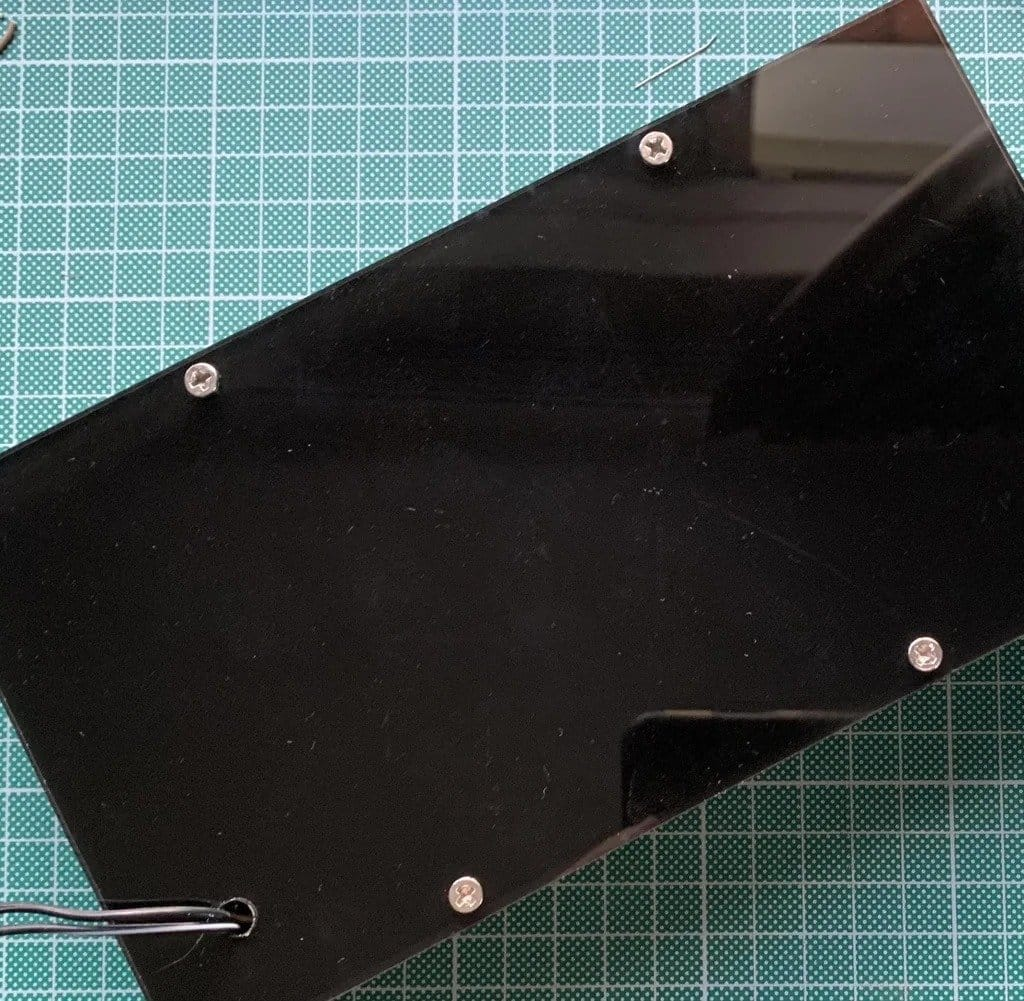Step four: PCB
For this project, the wizard used a previously designed PCB for another project. In this case, there are two 4511 chips on the PCB. The 4511 IC helps drive each display using binary code, which requires only four inputs to the display. As a result, the total number of I/O ports reaches 16. This allows you to use only one Arduino Nano board.
7Segment.brdStep five: circuit
You can not make a printed circuit board, but assemble a circuit on a perforated board. Port numbering and mapping is based on the code in the next step.
The first thing to keep in mind when installing is compactness. It is necessary that all these components can fit inside the case.
All wire connections must be insulated.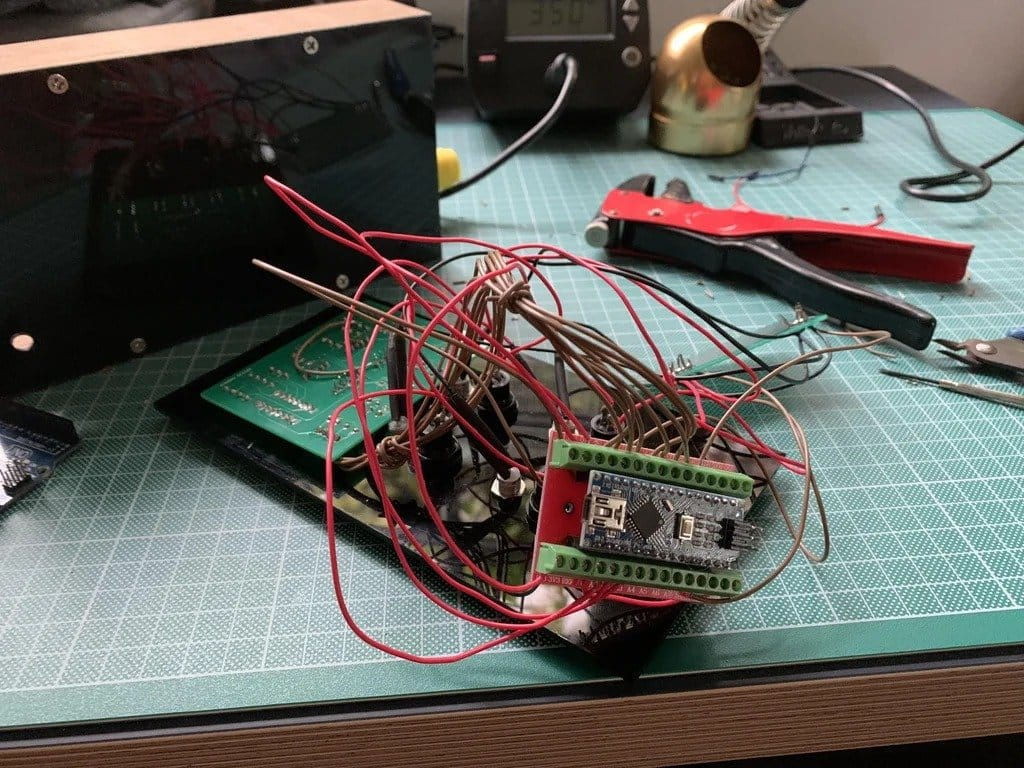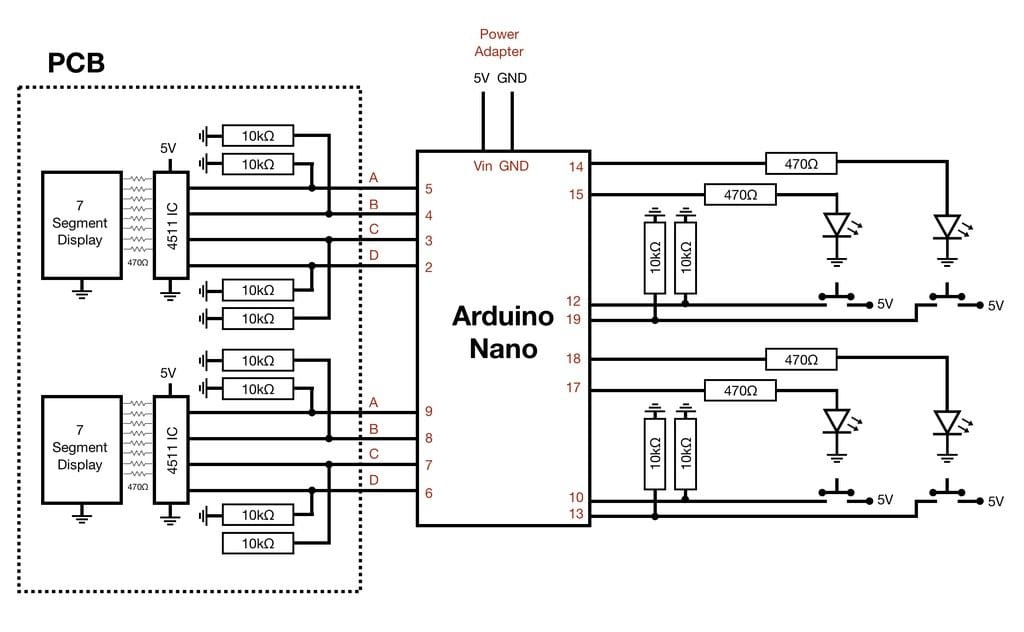Step six: code
Below is a file containing all the code for the project. All you need to do is upload the file, the Arduino IDE and upload the code to the Arduino Nano.
The code works like this
The program starts by creating a random list of 99 integers ranging from 1 to 4. The level is set to 1 and the first bit of the pattern is displayed. Once the user enters the expected number of keystrokes (for example, level 1 = 1 keystroke), the code checks the input against a list of 99 integers. If it matches, the code is repeated and the level is increased to 2 (and so on). If the input is incorrect, the code will restart.

` `//Written by Rehaan Irani, July 2021 int list & # 91; 99 & # 93 ;; int list2 & # 91; 99 & # 93 ;; int x = 1; int y = 0; int z = 0; int level = 1; int w; int c; int d; int e; int f; int g; int i; int j; int h; void setup () & # 123; //Declaring all our pins Serial.begin (9600); pinMode (2, OUTPUT); pinMode (3, OUTPUT); pinMode (4, OUTPUT); pinMode (5, OUTPUT); pinMode (6, OUTPUT); pinMode (7, OUTPUT); pinMode (8, OUTPUT); pinMode (9, OUTPUT); pinMode (14, OUTPUT); pinMode (15, OUTPUT); pinMode (17, OUTPUT); pinMode (18, OUTPUT); pinMode (10, INPUT); pinMode (11, INPUT); pinMode (12, INPUT); pinMode (13, INPUT); //This loop creates the random sequence of 99 random numbers between 1 and 4 for (int i = 0; i & lt; = 98; i ++) & # 123; list & # 91; i & # 93; = random (1, 5); }} //Reset function that we will call if the user inputs the incorrect pattern void (* resetFunc) (void) = 0; //Main Loop void loop () & # 123; //Condition set to ensure the user makes an input before the next sequence is shown if (x == 1) & # 123; //For loop displays the pattern on the LEDs - depends on the level for (int i = 0; i & lt; = level; i ++) & # 123; //Each if statement operates an LED which is triggered depending on if the integer is 1,2,3 or 4 if (list & # 91; i & # 93; == 1) & # 123; digitalWrite (15, HIGH); delay (1000); digitalWrite (15, LOW); } if (list & # 91; i & # 93; == 2) & # 123; digitalWrite (14, HIGH); delay (1000); digitalWrite (14, LOW); } if (list & # 91; i & # 93; == 3) & # 123; digitalWrite (17, HIGH); delay (1000); digitalWrite (17, LOW); } if (list & # 91; i & # 93; == 4) & # 123; digitalWrite (18, HIGH); delay (1000); digitalWrite (18, LOW); }} //If x is 0, the above code will not run again until x is set back to 1 x = 0; } /////////////////////////////////////////These IF statements record the users input depending on which button they press if (digitalRead (12) == 1) & # 123; list2 & # 91; y & # 93; = 1; y + = 1; delay (500); } if (digitalRead (18) == 1) & # 123; list2 & # 91; y & # 93; = 2; y + = 1; delay (500); } if (digitalRead (10) == 1) & # 123; list2 & # 91; y & # 93; = 3; y + = 1; delay (500); } if (digitalRead (13) == 1) & # 123; list2 & # 91; y & # 93; = 4; y + = 1; delay (500); } /////////////////////////////////////////////////////////////////This IF statements set the condition for running the check which only happens when the number of user inputs = level if (y == level + 1) & # 123; //This for loop checks each user input against the random list for (int i = 0; i & lt; = level; i ++) & # 123; //If the lists match, nothing happens, the program continues if (list2 & # 91; i & # 93; == list & # 91; i & # 93;) & # 123; Serial.print (& # 34; h & # 34;); //ELSE, if the lists do not match, the program calls on the reset function to restart from the beginning} else & # 123; Serial.print (& # 34; FUNCTION RESTART & # 34;); resetFunc (); } //x is set to one because the lists match and the next sequence can be shown} x = 1; //level is increased by one to display one more number on the random list level + = 1; //y is 0 to reset the users inputs y = 0; } //The rest of the code deals with displaying the level on the 7 segment displays //This part below separates numbers into its two digits (e.g. 43 = 4 and 3) int v; int randomNumber = level% 10; v = level - randomNumber; int randomNumber2 = v/10; //The first digit is converted to binary if (z & gt; 7) & # 123; //1st Digit Write f = HIGH; randomNumber = randomNumber% 8; } else & # 123; f = LOW; } if (randomNumber & gt; 3) & # 123; e = HIGH; randomNumber = randomNumber% 4; } else & # 123; e = LOW; } if (randomNumber & gt; 1) & # 123; d = HIGH; randomNumber = randomNumber% 2; } else & # 123; d = LOW; } if (randomNumber & gt; 0) & # 123; c = HIGH; randomNumber = randomNumber% 1; } else & # 123; c = LOW; } //The second digit is converted to binary if (randomNumber2 & gt; 7) & # 123; //2nd Digit Write j = HIGH; randomNumber2 = randomNumber2% 8; } else & # 123; j = LOW; } if (randomNumber2 & gt; 3) & # 123; i = HIGH; randomNumber2 = randomNumber2% 4; } else & # 123; i = LOW; } if (randomNumber2 & gt; 1) & # 123; h = HIGH; randomNumber2 = randomNumber2% 2; } else & # 123; h = LOW; } if (randomNumber2 & gt; 0) & # 123; g = HIGH; randomNumber2 = randomNumber2% 1; } else & # 123; g = LOW; } //The binary output is sent and displayed on the 7 segment displays digitalWrite (2, j); digitalWrite (3, i); digitalWrite (4, h); digitalWrite (5, g); digitalWrite (6, f); digitalWrite (7, e); digitalWrite (8, d); digitalWrite (9, c); } ` `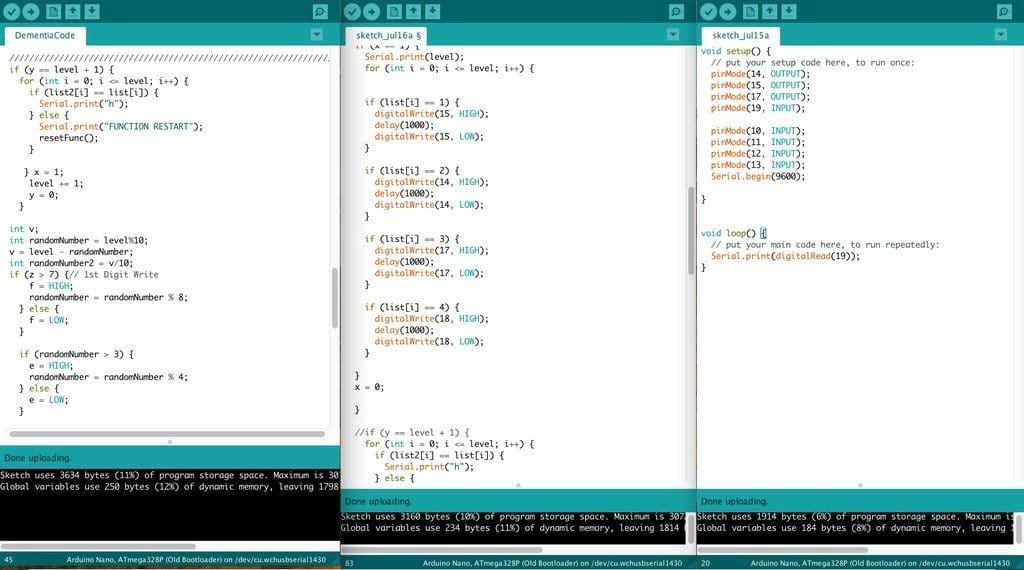Step seven: power supply
A 5 ​​V power adapter is used to power the device. The plus of the adapter is connected to Vin, and the minus to GND on the Arduino Nano.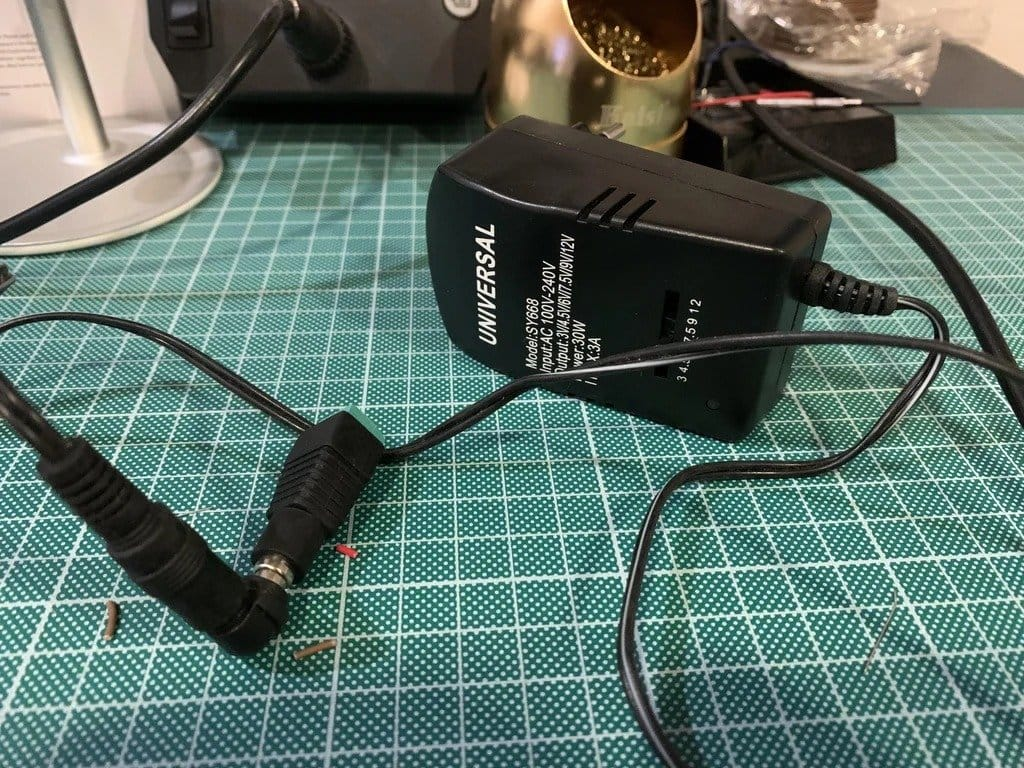Everything is ready.Source:

usamodelkina.ru